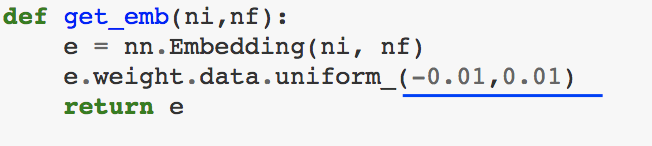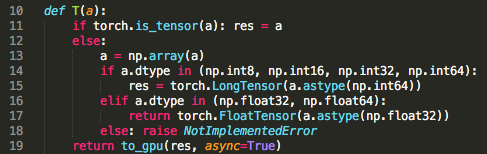# Lesson 5 In-Class Discussion

At the moment there isn’t separate datasets of this type for regression vs classification, so it’s up to you to ensure your dependent is of the right type. If we cast it to float always (as I used to do) it doesn’t work for classification.

I actually think 1e-2 looks about right here. It’s dropping quickly at that point.

That’s not what we want if we use SGDR (which I think we always should) - we want a max learning rate which does cause a bit of a jump!

2 Likes

That isn’t used unless you add a param to explicitly enable it.

1 Like

Thanks for the explanation JeremyAhh my bad. I saw the `movielens` notebook using `optim.Adam` call and `fit` using `weight decay` and `weight decay scheduler`. Doesn’t this mean it’s using `AdamW`? Or do we need another param to enable this? (I might have a totally incorrect understanding of AdamW at this point).

@A_TF57 You need to set `use_wd_sched=True` to use AdamW, check it here: New AdamW optimizer now available

3 Likes

Why the initialized weights are little different when we are doing `collaborative filtering from scratch` in cases when we added bias and we didn’t add bias. And is this initialization random or based on some logic?

Without bias --> initialized as uniform distribution b/w 0 and 0.05

With bias. initialized as uniform distribution b/w -0.01 and 0.01ThanksTo answer the randomness question, check up `uniform()` in the pytorch docs and tell us what you find out. And see if you can remember what `_` suffix does…As to why I used those init values - well honestly I didn’t spend much time thinking about it and just picked something roughly reasonable.

Oh. I meant, I understood that `e.weight.data.uniform_` is replacing and initializing weights in embedding matrix with random uniform distribution between -0.01 to 0.01.

My point of asking `random or with logic` was how to chose a and b in `uniform(a,b)`. Why not uniform distribution b/w -1 to 1 OR -5 to 5 OR 0.5 to 0.75?

So as you say, you just picked something roughly reasonable. I get it, so it should’t matter right? We can chose any range (something reasonable though. i.e. not so high or so low range)

Ah apologies for misunderstanding.

Yes it does matter, a lot! See the first chart here: https://intoli.com/blog/neural-network-initialization/

Generally we use Kaiming He or Xavier Glorot initialization. Eg see https://prateekvjoshi.com/2016/03/29/understanding-xavier-initialization-in-deep-neural-networks/

The goal is to pick an init such that all the layers have approx mean 0 stdev 1

3 Likes

Oh. I get it now. Xavier Initialization link you shared has clear and nice explanation. So if we are taking weights from normal distribution, our aim is to maintain same variance as we pass through each layer and hence we take weights from a random normal distribution with variance = 1/N(input neurons). (for `Xavier`)

1 Like

I was trying to connect some dots related to PMF, SVD, PCA and Embeddings and got confused.

So, in lecture 5, Jeremy said that the method of dot product of movie embedding matrix and user embedding matrix is actually Probabilistic Matrix Factorization (PMF) and he also said that those matrices are literally just Embeddings.

Then here, it is written that SVD is actually equal to PMF when additional bias is added. And we already know from linear algebra that PCA (Principal Component Analysis) is an application of SVD .

So it points out that embedding matrix is somewhat related to PCA. (although I remember that I read somewhere that these are very different). Which of above stated points is wrong then? (PMF => Embeddings => SVD => PCA)

`The shared link is from Surprise package which is well documented package for collaborative filtering`

I’m pretty sure that link is wrong. SVD requires an orthogonal factorization. Thanks for the interesting question!

1 Like

I was reviewing lesson 5 and noticed something.

Here, @jeremy mentions that since we did not say .cuda(), these tensors are sitting on CPU and not on GPU:

But looking at a code, it seems like they are on GPU if `torch.cuda.is_available()` is true.Does that sound accurate? Or am I missing something?

Thank you!

Yes, this is correct. If pytorch detects that cuda is available, it’ll automatically use it. If not, the tensors will be numpy arrays by default.

1 Like

Great! Thank you,BrianSuggested mods for model.py, this notebook does not specify callbacks to the model so it defaults to none, which is a problem I’ve seen before in my own notebooks. Please make the specified changes below to model.fit.

``````def fit(model, data, epochs, opt, crit, metrics=None, callbacks=None, **kwargs):
""" Fits a model

Arguments:
model (model): any pytorch module
net = to_gpu(net)
data (ModelData): see ModelData class and subclasses
epochs(int): number of epochs
crit: loss function to optimize. Example: F.cross_entropy
"""
stepper = Stepper(model, opt, crit, **kwargs)
metrics = metrics or []
callbacks = callbacks or []
avg_mom=0.98
batch_num,avg_loss=0,0.
if callbacks and hasattr(callbacks,'on_train_begin'):
for cb in callbacks:
cb.on_train_begin()
names = ["epoch", "trn_loss", "val_loss"] + [f.__name__ for f in metrics]
layout = "{!s:10} " * len(names)

num_batch = len(data.trn_dl)
if epochs<1:
num_batch = int(num_batch*epochs)
epochs = 1

for epoch in tnrange(epochs, desc='Epoch'):
stepper.reset(True)
t = tqdm(iter(data.trn_dl), leave=False, total=num_batch)
i = 0
for (*x,y) in t:
batch_num += 1
if callbacks and hasattr(callbacks,'on_batch_begin'):
for cb in callbacks: cb.on_batch_begin()
loss = stepper.step(V(x),V(y))
avg_loss = avg_loss * avg_mom + loss * (1-avg_mom)
debias_loss = avg_loss / (1 - avg_mom**batch_num)
t.set_postfix(loss=debias_loss)
stop=False
if callbacks and (hasattr(callbacks,'on_batch_end') or hasattr(callbacks,'stop')):
for cb in callbacks: stop = stop or cb.on_batch_end(debias_loss)
if stop: return
if i>num_batch: break
i += 1

vals = validate(stepper, data.val_dl, metrics)
if epoch == 0: print(layout.format(*names))
print_stats(epoch, [debias_loss] + vals)
stop=False
if callbacks and (hasattr(callbacks,'on_epoch_end') or hasattr(callbacks,'stop')):
for cb in callbacks: stop = stop or cb.on_epoch_end(vals)
if stop: break

if callbacks and hasattr(callbacks,'on_train_end'):
for cb in callbacks: cb.on_train_end()
return vals``````

Is there a good way to do collaborative filtering when the level is implicit? ie. we only have a positive label, the missing part we don’t know what the label is (or we can think of it as weakly negative)?

Chris, was your problem resolved because I have still the same issue (the one where you reported a bug when using learn.fit)? Thanks

You can do something called negative sampling. You can sample (user, item) pair at random and give them negative label (zero). It is discussed in many papers. (https://arxiv.org/abs/1402.3722)